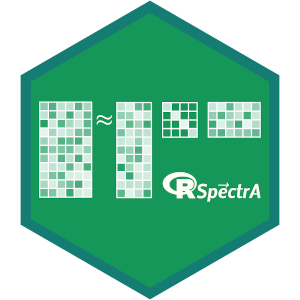# README.md In RSpectra: Solvers for Large-Scale Eigenvalue and SVD Problems

## Solvers for Large Scale Eigenvalue and SVD Problems### Introduction

RSpectra is an R interface to the Spectra library. It is typically used to compute a few eigenvalues/vectors of an `n` by `n` matrix, e.g., the `k` largest eigen values, which is usually more efficient than `eigen()` if `k << n`.

Currently this package provides the function `eigs()` for eigenvalue/eigenvector problems, and `svds()` for truncated SVD. Different matrix types in R, including sparse matrices, are supported. Below is a list of implemented ones:

• `matrix` (defined in base R)
• `dgeMatrix` (defined in Matrix package, for general matrices)
• `dgCMatrix` (defined in Matrix package, for column oriented sparse matrices)
• `dgRMatrix` (defined in Matrix package, for row oriented sparse matrices)
• `dsyMatrix` (defined in Matrix package, for symmetric matrices)
• `dsCMatrix` (defined in Matrix package, for symmetric column oriented sparse matrices)
• `dsRMatrix` (defined in Matrix package, for symmetric row oriented sparse matrices)
• `function` (implicitly specify the matrix by providing a function that calculates matrix product `A %*% x`)

### Examples

We first generate some matrices:

``````library(Matrix)
n = 20
k = 5

set.seed(111)
A1 = matrix(rnorm(n^2), n)  ## class "matrix"
A2 = Matrix(A1)             ## class "dgeMatrix"
``````

General matrices have complex eigenvalues:

``````eigs(A1, k)
eigs(A2, k, opts = list(retvec = FALSE))  ## eigenvalues only
``````

RSpectra also works on sparse matrices:

``````A1[sample(n^2, n^2 / 2)] = 0
A3 = as(A1, "dgCMatrix")
A4 = as(A1, "dgRMatrix")

eigs(A3, k)
eigs(A4, k)
``````

Function interface is also supported:

``````f = function(x, args)
{
as.numeric(args %*% x)
}
eigs(f, k, n = n, args = A3)
``````

Symmetric matrices have real eigenvalues.

``````A5 = crossprod(A1)
eigs_sym(A5, k)
``````

To find the smallest (in absolute value) `k` eigenvalues of `A5`, we have two approaches:

``````eigs_sym(A5, k, which = "SM")
eigs_sym(A5, k, sigma = 0)
``````

The results should be the same, but the latter method is far more stable on large matrices.

For SVD problems, you can specify the number of singular values (`k`), number of left singular vectors (`nu`) and number of right singular vectors(`nv`).

``````m = 100
n = 20
k = 5
set.seed(111)
A = matrix(rnorm(m * n), m)

svds(A, k)
svds(t(A), k, nu = 0, nv = 3)
``````

Similar to `eigs()`, `svds()` supports sparse matrices:

``````A[sample(m * n, m * n / 2)] = 0
Asp1 = as(A, "dgCMatrix")
Asp2 = as(A, "dgRMatrix")

svds(Asp1, k)
svds(Asp2, k, nu = 0, nv = 0)
``````

and function interface

``````f = function(x, args)
{
as.numeric(args %*% x)
}
g = function(x, args)
{
as.numeric(crossprod(args, x))
}
svds(f, k, Atrans = g, dim = c(m, n), args = Asp1)
``````

## Try the RSpectra package in your browser

Any scripts or data that you put into this service are public.

RSpectra documentation built on Jan. 11, 2020, 9:33 a.m.